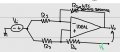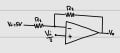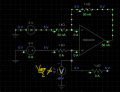# Voltage at the opamp inputs

#### kalemaxon89

Joined Oct 12, 2022
159
I have a doubt about the two green voltages in the opamp shown.Let's assume an ideal opamp and all the resistors are equal
I noticed in the drawing that the green point V4 I indicated is a bit ambiguous, it is NOT an applied voltage .. it's simply the terminal to which I would connect the '-' of the voltmeter measuring Vo .. while the '+' is Vo. R4 is floating.

In lecture V+ was calculated as Vin/2=2.5V and the (simplified) equiavalent circuit is as follows:I tried to simulate the circuit on Everycircuit (app which I don't know how reliable it can be) and found this result:1) Initially, I thought of performing a voltage divider since the 2 resistors are in series (and therefore the same current flows, although ideally zero in this case) .. in fact V+=Vi*1k/(1k+1k)=2.5V

2) Then, by watching the simulation, I had this doubt: there is no current circulating on the R3 and R4 as it is an open circuit, so there can be no voltage across each resistor. Why V+is not equal to 5V ?? (as the simulation shows).
Why don't I see 2.5V in simulation ??

Last edited:

#### crutschow

Joined Mar 14, 2008
32,064
Obviously with all the input resistors tied to 5V there would be no current through any of the resistors as there is no voltage across them.
For the voltage at the op amp (+) input to be 2.5V, the right bottom (unlabeled) resistor must be connected to ground.

Please label you resistor as it makes commenting about them much easier.

#### WBahn

Joined Mar 31, 2012
28,492
You've got two signals labeled "Vi" in your circuit. That implies that they are the same signal -- not two different signals that happen to have the same value, but the same signal such that they must ALWAYS be the same value. But your simulation has them as two different signals. Which is it?

You talk about Vref, but your circuit doesn't have a Vref in it. What, and where, is Vref?

Your circuit shows the bottom-right resistor dangling. Why is it even there if it's not connected to anything?

Are you sure your notes accurately reflect the circuit that was used in lecture? My guess is that the bottom input is not Vi, but rather Vref. Also, I'm guessing that the right side of the bottom-right resistor is supposed to be connected to ground. That is what will make the voltage at the non-inverting input of the opamp equal to Vref/2.

#### kalemaxon89

Joined Oct 12, 2022
159
You've got two signals labeled "Vi" in your circuit. That implies that they are the same signal -- not two different signals that happen to have the same value, but the same signal such that they must ALWAYS be the same value. But your simulation has them as two different signals. Which is it?

You talk about Vref, but your circuit doesn't have a Vref in it. What, and where, is Vref?

Your circuit shows the bottom-right resistor dangling. Why is it even there if it's not connected to anything?

Are you sure your notes accurately reflect the circuit that was used in lecture? My guess is that the bottom input is not Vi, but rather Vref. Also, I'm guessing that the right side of the bottom-right resistor is supposed to be connected to ground. That is what will make the voltage at the non-inverting input of the opamp equal to Vref/2.
Sorry for the mistake, I've just updated the figures in the post.

I'm absolutely sure that I've copied correctly the schematic shown in the whiteboard at the lesson. The R4 resistor is floating and we want to know the voltage between Vo and the green V4 potential I've indicate in the figure. The feedback resistor R2 should rapresent a NTC sensor resistor whose resistance changes as a function of temperature.

I am not interested in the steps to find Vo etc. ... but I do not understand why the first step to solve the circuit was to simplify the positive input with 2.5V

#### kalemaxon89

Joined Oct 12, 2022
159
Obviously with all the input resistors tied to 5V there would be no current through any of the resistors as there is no voltage across them.
For the voltage at the op amp (+) input to be 2.5V, the right bottom (unlabeled) resistor must be connected to ground.

Please label you resistor as it makes commenting about them much easier.
I've just updated the figures in the post.

#### WBahn

Joined Mar 31, 2012
28,492
Sorry for the mistake, I've just updated the figures in the post.

I'm absolutely sure that I've copied correctly the schematic shown in the whiteboard at the lesson. The R4 resistor is floating and we want to know the voltage between Vo and the green V4 potential I've indicate in the figure. The feedback resistor R2 should rapresent a NTC sensor resistor whose resistance changes as a function of temperature.

I am not interested in the steps to find Vo etc. ... but I do not understand why the first step to solve the circuit was to simplify the positive input with 2.5V
For the circuit as you now have it, the voltage at the non-inverting input will NOT be Vin/2. If one end of R4 is floating, then no current can flow in it and, hence, no voltage drop across it. Similarly, only the opamp's input bias current will flow in R3 (which you are assuming to be zero since you are assuming an ideal opamp).

#### crutschow

Joined Mar 14, 2008
32,064
I've just updated the figures in the post.
Still incorrect.
The bottom right resistor should go to ground.

•kalemaxon89

#### MrAl

Joined Jun 17, 2014
10,087
I have a doubt about the two green voltages in the opamp shown.
View attachment 290239
Let's assume an ideal opamp and all the resistors are equal
In lecture V+ was calculated as Vin/2=2.5V and the (simplified) equiavalent circuit is as follows:
View attachment 290238
I tried to simulate the circuit on Everycircuit (app which I don't know how reliable it can be) and found this result:
View attachment 290213

1) Initially, I thought of performing a voltage divider since the 2 resistors are in series (and therefore the same current flows, although ideally zero in this case) .. in fact V+=Vi*1k/(1k+1k)=2.5V

2) Then, by watching the simulation, I had this doubt: there is no current circulating on the R3 and R4 as it is an open circuit, so there can be no voltage across each resistor. Why V+is not equal to 5V ?? (as the simulation shows).
Why don't I see 2.5V in simulation ??
Hello,

Are you stating that V4 is another externally applied voltage in addition to Vi ?
If so, the circuit is just an inverting amplifier with adjustable offset, sort of. However, if you do a full analysis i think you will get a little surprise as to what the output is for any Vi and any V4 with all resistors equal including the NTC thermistor.

They may be assuming Vi/2 for the condition of V4=0 only, along with R4=R3 and maybe some other assumptions too. That may be the first step they want you to see. At a later date they may ask you to set V4 to another voltage.

#### kalemaxon89

Joined Oct 12, 2022
159
For the circuit as you now have it, the voltage at the non-inverting input will NOT be Vin/2. If one end of R4 is floating, then no current can flow in it and, hence, no voltage drop across it. Similarly, only the opamp's input bias current will flow in R3 (which you are assuming to be zero since you are assuming an ideal opamp).
Hello,

Are you stating that V4 is another externally applied voltage in addition to Vi ?
If so, the circuit is just an inverting amplifier with adjustable offset, sort of. However, if you do a full analysis i think you will get a little surprise as to what the output is for any Vi and any V4 with all resistors equal including the NTC thermistor.

They may be assuming Vi/2 for the condition of V4=0 only, along with R4=R3 and maybe some other assumptions too. That may be the first step they want you to see. At a later date they may ask you to set V4 to another voltage.
I noticed in the drawing that the green point V4 I indicated is a bit ambiguous, it is NOT an applied voltage .. it's simply the terminal to which I would connect the '-' of the voltmeter measuring Vo .. while the '+' is Vo. R4 is floating.

#### MrAl

Joined Jun 17, 2014
10,087
I noticed in the drawing that the green point V4 I indicated is a bit ambiguous, it is NOT an applied voltage .. it's simply the terminal to which I would connect the '-' of the voltmeter measuring Vo .. while the '+' is Vo. R4 is floating.
Oh ok, but that is a bit unusual unless there was supposed to be some variance with load from Vo to V4.
If that is the case, then Vi/2 does not apply as the non inverting input will equal Vi, unless there is a load from Vo to V4 as mentioned.

Was there any specific application noted for this circuit?

•kalemaxon89

#### kalemaxon89

Joined Oct 12, 2022
159
Oh ok, ma questo è un po 'insolito a meno che non ci fosse qualche variazione con il carico da Vo a V4.
Se questo è il caso, allora Vi/2 non si applica in quanto l'ingresso non invertente sarà uguale a Vi, a meno che non ci sia un carico da Vo a V4 come menzionato.

C'è stata qualche applicazione specifica nota per questo circuito?
R2 is the resistance of a NTC sensor that varies with the temperature. In fact, Vo(T) voltage is a function of temperature

#### crutschow

Joined Mar 14, 2008
32,064
R4 is floating.
That is likely incorrect as it would seem R4 should be grounded.
Since the voltage difference across the two op amp inputs is essentially zero, connecting R4 to ground will generate a constant current through the R2 NTC sensor equal to Vin / 2R1.
The output voltage will then be directly proportional to the resistance of NTC R2 but inverted (the voltage becomes more positive as R2 decreases, indicating a temperature increase of R2).
The output will be 0V when R2 = R4 (assuming R1 = R3). (You may want to select R4 so give an output of zero at zero degrees)

Seem correct?

#### MrAl

Joined Jun 17, 2014
10,087
R2 is the resistance of a NTC sensor that varies with the temperature. In fact, Vo(T) voltage is a function of temperature
Hi,

That's funny, i studied the Spanish language but didn't realize i speak it without realizing it sometimes (ha ha ha).

Something still does not seem right with this circuit as others have pointed out too. Why measure the voltage like that, especially when it is through a resistor (R4) that does nothing when it is connected the way you say it should be connected.

One thing we do know, and that is that the output is supposed to be a function of temperature. The question now is, what function would that be. If we do this in a more normal way we can come up with some good results. It still looks like V4 should be connected to a reference voltage of some value to be able to get a better output function with temperature. We could look into this. Remember the thermistor is non linear with temperature, in fact, sort of logarithmic, and adding or subtracting a constant value allows us to get a more linear result within a given range at a given target temperature point.

I might look into this also as i have not done this in a long time now and i am working on something the past few months were i wanted to add approximate temperature measurements to the mix. That's if i don't decide to go completely digital.

•kalemaxon89

#### WBahn

Joined Mar 31, 2012
28,492
I'm absolutely sure that I've copied correctly the schematic shown in the whiteboard at the lesson. The R4 resistor is floating and we want to know the voltage between Vo and the green V4 potential I've indicate in the figure. The feedback resistor R2 should rapresent a NTC sensor resistor whose resistance changes as a function of temperature.
This doesn't ring true.

#### crutschow

Joined Mar 14, 2008
32,064
The R4 resistor is floating
Makes no sense to have a floating resistor as it has no current flowing through it and therefore does nothing in the circuit, thus it can be removed with no change in circuit operation.

#### kalemaxon89

Joined Oct 12, 2022
159
Makes no sense to have a floating resistor as it has no current flowing through it and therefore does nothing in the circuit, thus it can be removed with no change in circuit operation.
I think so too

#### kalemaxon89

Joined Oct 12, 2022
159
This doesn't ring true.

This doesn't ring true.

I mentioned Vref at first but I noticed that I had written Vi in the circuit, so I corrected myself.
There is only one input voltage in the circuit and that is Vi.

Probably at school the teacher forgot to ground R4. I'll ask.

#### kalemaxon89

Joined Oct 12, 2022
159
Hi,

That's funny, i studied the Spanish language but didn't realize i speak it without realizing it sometimes (ha ha ha).

Something still does not seem right with this circuit as others have pointed out too. Why measure the voltage like that, especially when it is through a resistor (R4) that does nothing when it is connected the way you say it should be connected.

One thing we do know, and that is that the output is supposed to be a function of temperature. The question now is, what function would that be. If we do this in a more normal way we can come up with some good results. It still looks like V4 should be connected to a reference voltage of some value to be able to get a better output function with temperature. We could look into this. Remember the thermistor is non linear with temperature, in fact, sort of logarithmic, and adding or subtracting a constant value allows us to get a more linear result within a given range at a given target temperature point.

I might look into this also as i have not done this in a long time now and i am working on something the past few months were i wanted to add approximate temperature measurements to the mix. That's if i don't decide to go completely digital.
Probably at school the teacher forgot to ground R4. I'll ask.

#### MrAl

Joined Jun 17, 2014
10,087
Probably at school the teacher forgot to ground R4. I'll ask.
Hi,

That may be true, but what i was hinting at was that for example if you apply a voltage where V4 is you can adjust the output to get a negative value for minus temperatures and a positive output value for positive temperatures. Between Vi and V4 that way you may be able to get some sort of calibration, which is always a consideration in these analog type thermistor temperature measurement circuits. Once you get some degree of calibration and offset in these kinds of circuits you can often get some reasonable linarity even though it won't be perfect. That's what gave me the impression that V4 might be a voltage source, if that is what they wanted to do with this circuit that is.
It could be a simpler exercise of course that's always a possibility.

•kalemaxon89